# Econometric Modelling Introduction To examine some econometric results

• Slides: 29Econometric ModellingIntroduction • To examine some econometric results from various financial models • To use the results to determine levels of significance of the variables and whether the results fit theory • To use the results for testing specific restrictions. • Suggest some potential problems when assessing model results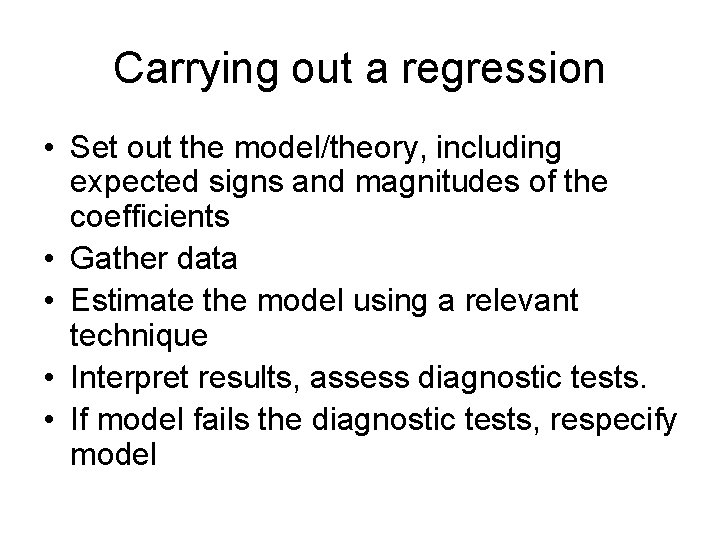Carrying out a regression • Set out the model/theory, including expected signs and magnitudes of the coefficients • Gather data • Estimate the model using a relevant technique • Interpret results, assess diagnostic tests. • If model fails the diagnostic tests, respecify model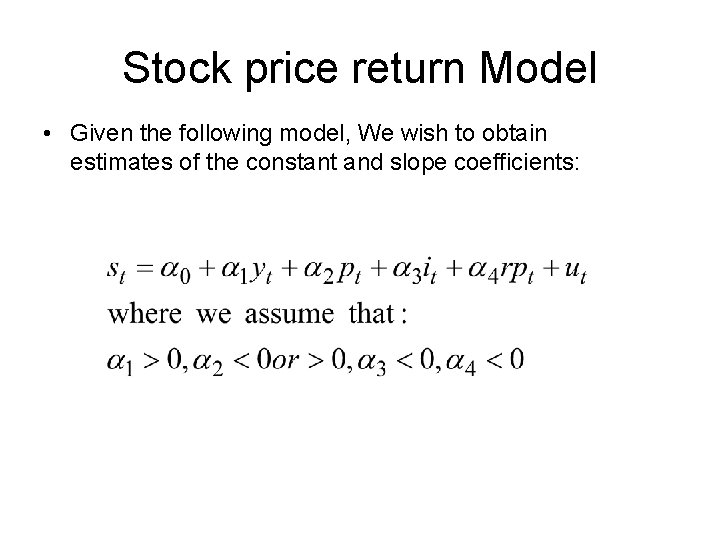Stock price return Model • Given the following model, We wish to obtain estimates of the constant and slope coefficients: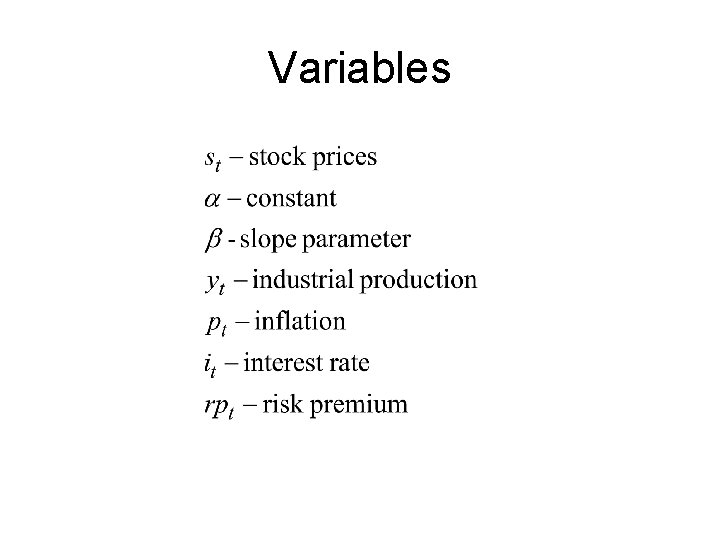Variables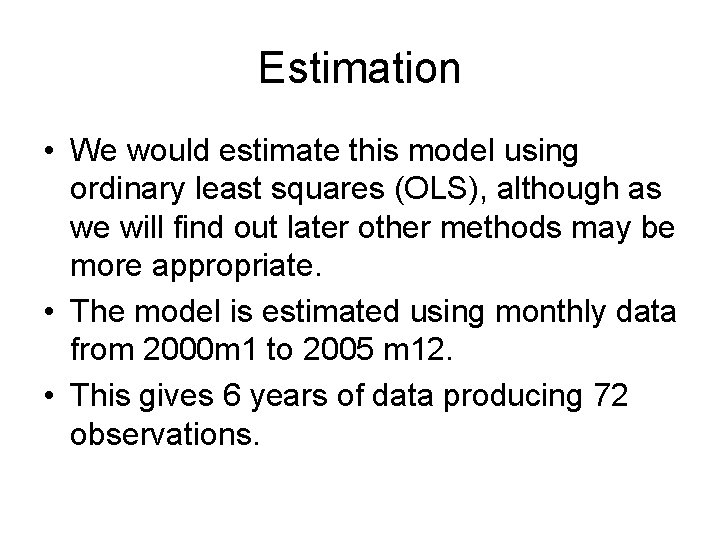Estimation • We would estimate this model using ordinary least squares (OLS), although as we will find out later other methods may be more appropriate. • The model is estimated using monthly data from 2000 m 1 to 2005 m 12. • This gives 6 years of data producing 72 observations.Results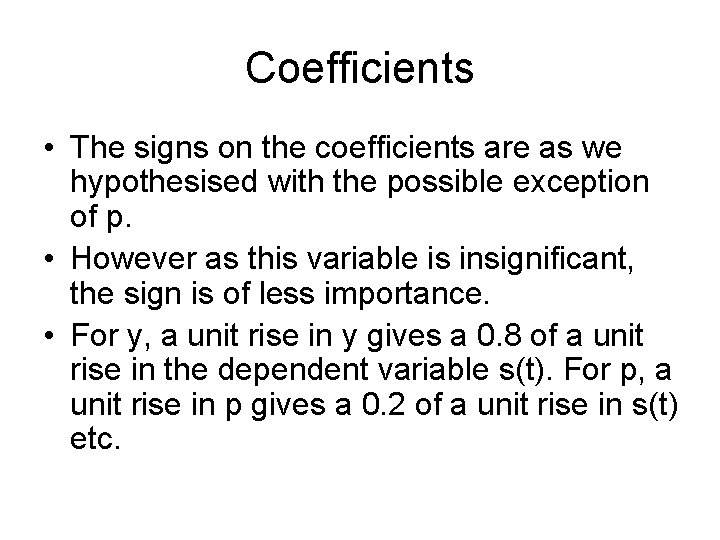Coefficients • The signs on the coefficients are as we hypothesised with the possible exception of p. • However as this variable is insignificant, the sign is of less importance. • For y, a unit rise in y gives a 0. 8 of a unit rise in the dependent variable s(t). For p, a unit rise in p gives a 0. 2 of a unit rise in s(t) etc.T-statistics • Firstly test if the 4 variables are individually different to 0, using the t-test (we usually ignore the constant) • E. g. y: 0. 8 -0/0. 2=4 • Critical value is 2. 000 (5%) (72 -5 degrees of freedom, 60 d of f is nearest in tables) • As 4>2 we reject the null hypothesis that y=0, therefore y is said to be significantly different to 0. • The t-statistics for p: 1, i: 10 and rp: 2. 333, we conclude that y, i and rp are significant and p is insignificant. • This result would suggest we might consider removing p from our model.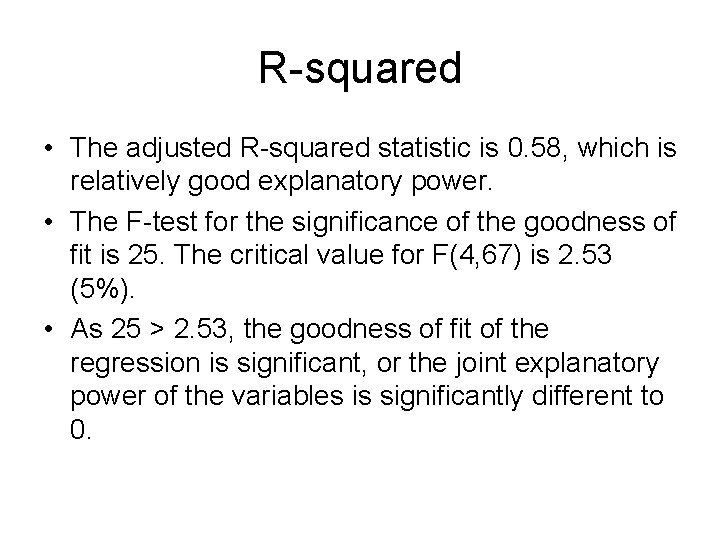R-squared • The adjusted R-squared statistic is 0. 58, which is relatively good explanatory power. • The F-test for the significance of the goodness of fit is 25. The critical value for F(4, 67) is 2. 53 (5%). • As 25 > 2. 53, the goodness of fit of the regression is significant, or the joint explanatory power of the variables is significantly different to 0.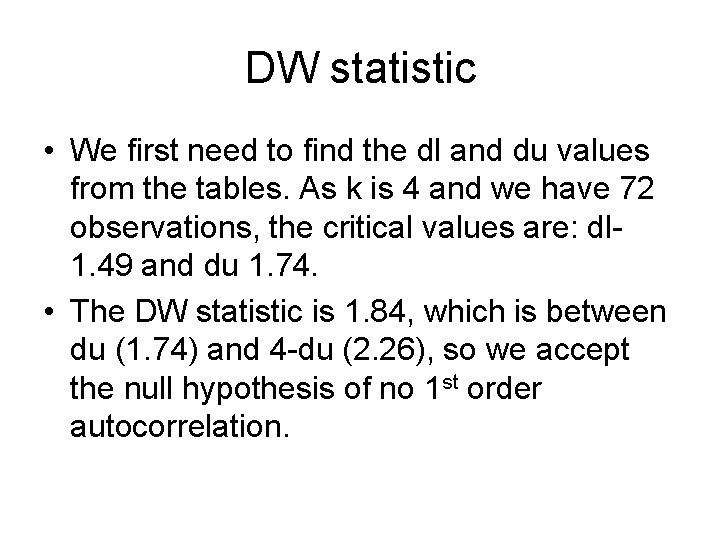DW statistic • We first need to find the dl and du values from the tables. As k is 4 and we have 72 observations, the critical values are: dl 1. 49 and du 1. 74. • The DW statistic is 1. 84, which is between du (1. 74) and 4 -du (2. 26), so we accept the null hypothesis of no 1 st order autocorrelation.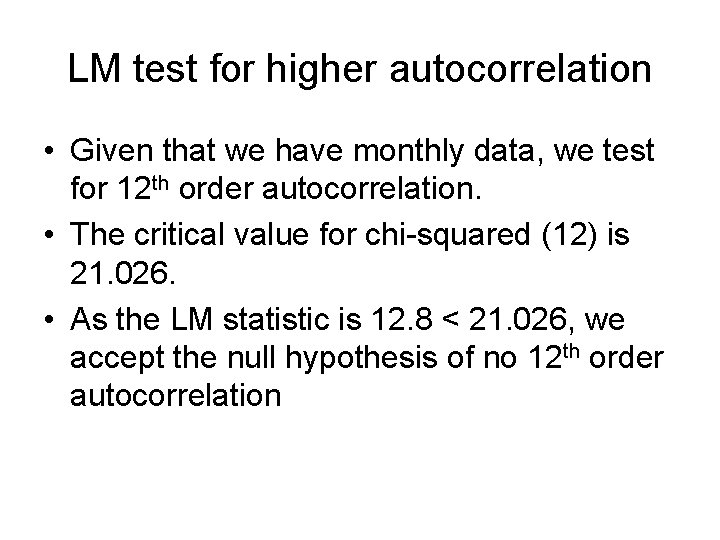LM test for higher autocorrelation • Given that we have monthly data, we test for 12 th order autocorrelation. • The critical value for chi-squared (12) is 21. 026. • As the LM statistic is 12. 8 < 21. 026, we accept the null hypothesis of no 12 th order autocorrelationWhite’s test for heteroskedasticity • This follows a chi-squared distribution with 14 degrees of freedom (including cross product terms) • The critical value with 14 degrees of freedom is: 23. 685 using the chi-squared tables • As 15. 2 < 23. 685, we accept the null hypothesis that there is no heteroskedasticity.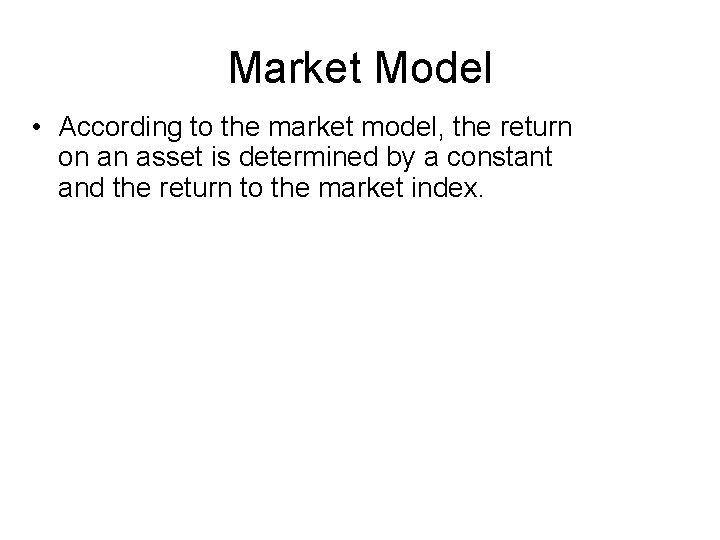Market Model • According to the market model, the return on an asset is determined by a constant and the return to the market index.Market Model • As before we would wish to run an OLS regression, then interpret the coefficient, tstatistics and various diagnostic tests for autocorrelation and heteroskedasticity. • In this model we would expect β > 0, the closer to 1, the closer asset i follows the market index. • If we have 100 days of daily data for the regression, we get the following result: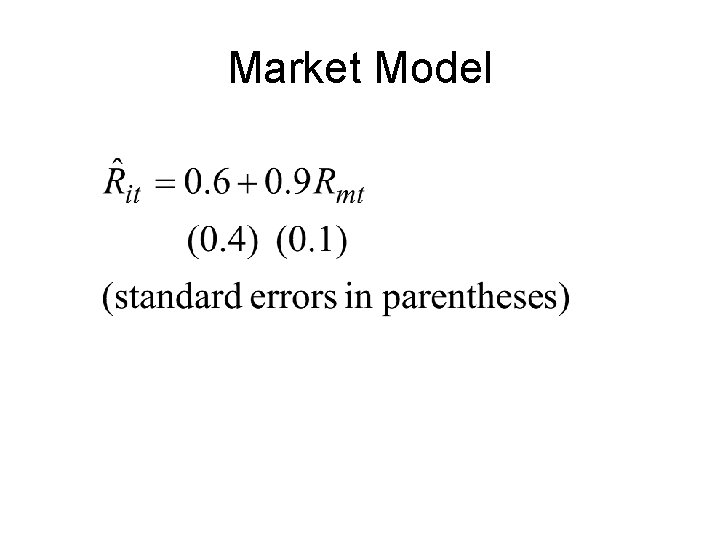Market ModelMarket Model • The result shows that a unit rise in the market produces a 0. 9 of a unit rise in asset i. This suggests this asset closely follows the market and would be considered safe. • The t-statistic shows that the market index is significant, 0. 9 -0/0. 1=9. critical value is 1. 98. As 9 > 1. 98, we reject the null hypothesis and say that the market has a significant effect on asset i. • The usual diagnostic tests would have been produced and interpreted as before.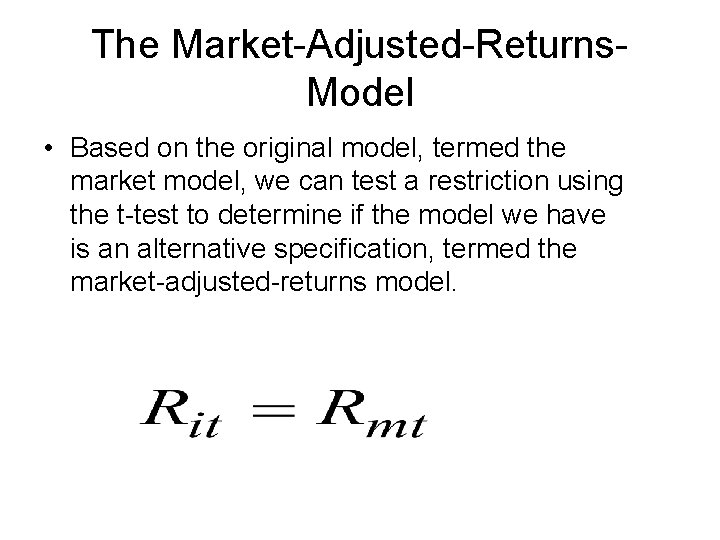The Market-Adjusted-Returns. Model • Based on the original model, termed the market model, we can test a restriction using the t-test to determine if the model we have is an alternative specification, termed the market-adjusted-returns model.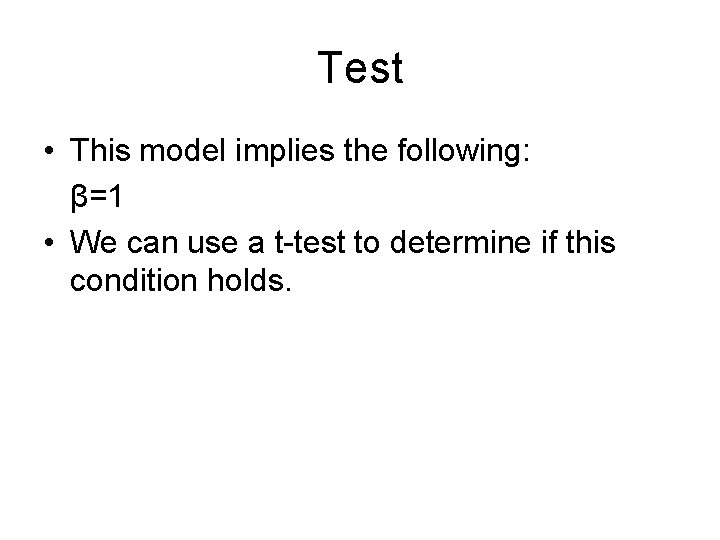Test • This model implies the following: β=1 • We can use a t-test to determine if this condition holds.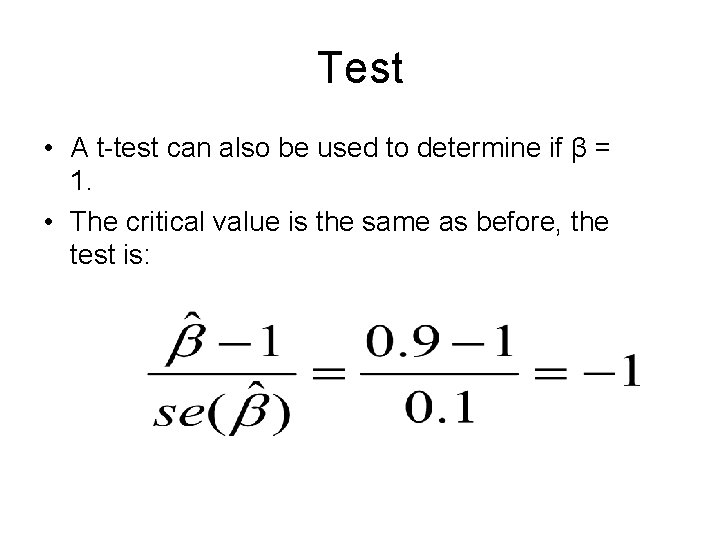Test • A t-test can also be used to determine if β = 1. • The critical value is the same as before, the test is:Test • As 1< 2 (ignore the sign the t-statistic is an absolute value), we fail to reject the null hypothesis and conclude that the market adjusted model applies. • In this case the hypothesis is:F-test of a restriction • The main use of this F-test is to determine if a group of explanatory variables are jointly equal to 0. • However we can test alternative theories or restrictions • The most common restriction is that 2 or more explanatory variables sum to 1. • The best example of this is the Cobb. Douglas production function.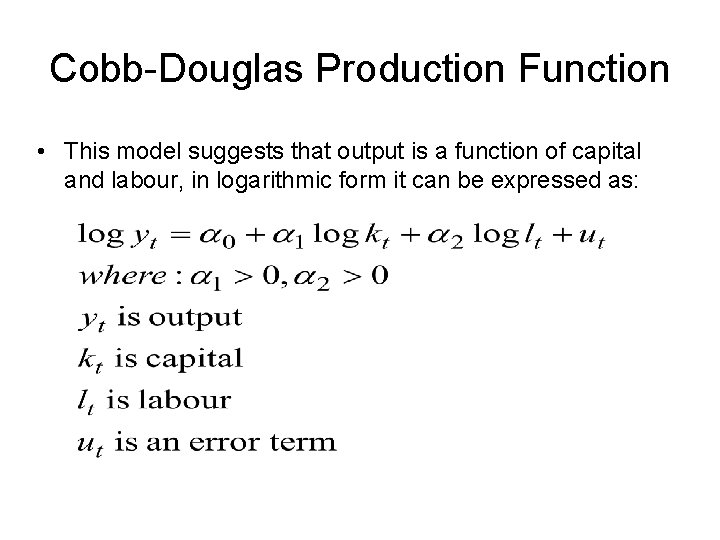Cobb-Douglas Production Function • This model suggests that output is a function of capital and labour, in logarithmic form it can be expressed as: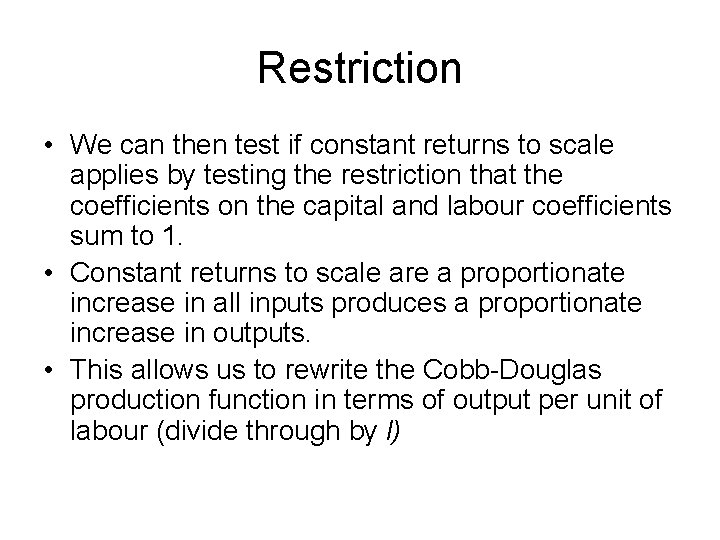Restriction • We can then test if constant returns to scale applies by testing the restriction that the coefficients on the capital and labour coefficients sum to 1. • Constant returns to scale are a proportionate increase in all inputs produces a proportionate increase in outputs. • This allows us to rewrite the Cobb-Douglas production function in terms of output per unit of labour (divide through by l)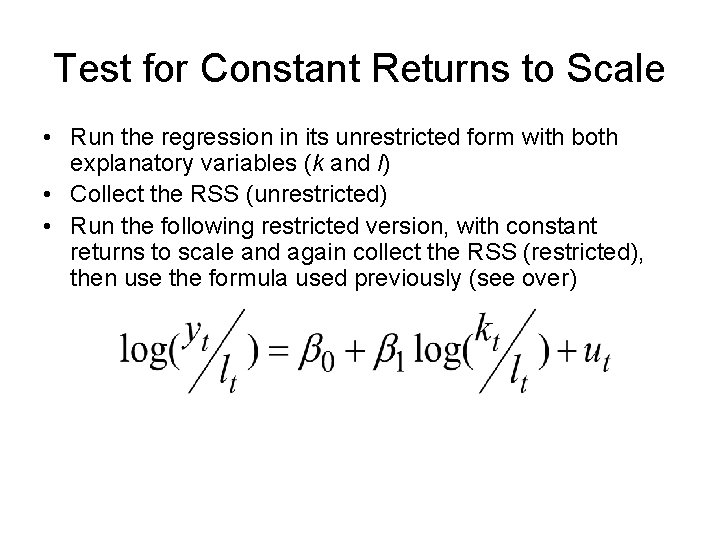Test for Constant Returns to Scale • Run the regression in its unrestricted form with both explanatory variables (k and l) • Collect the RSS (unrestricted) • Run the following restricted version, with constant returns to scale and again collect the RSS (restricted), then use the formula used previously (see over)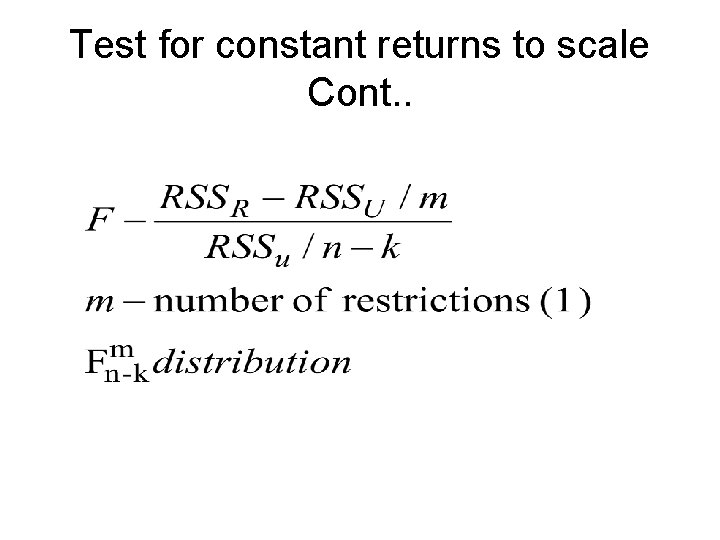Test for constant returns to scale Cont. .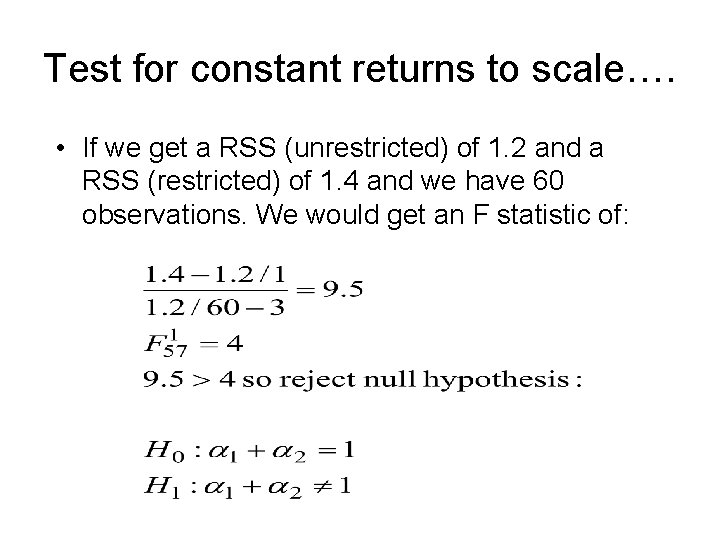Test for constant returns to scale…. • If we get a RSS (unrestricted) of 1. 2 and a RSS (restricted) of 1. 4 and we have 60 observations. We would get an F statistic of:Constant returns to scale… • We would reject the null hypothesis of constant returns to scale, therefore we would use the unrestricted model with capital and labour included separately. The null hypothesis is: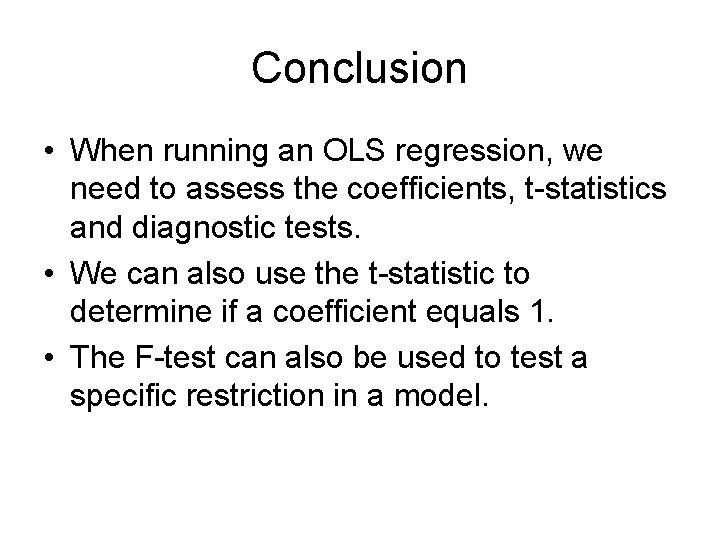Conclusion • When running an OLS regression, we need to assess the coefficients, t-statistics and diagnostic tests. • We can also use the t-statistic to determine if a coefficient equals 1. • The F-test can also be used to test a specific restriction in a model.